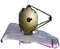# JWST ETC Cosmic Ray ImplementationThe JWST Exposure Time Calculator (ETC) accounts for the effects of cosmic rays in calculations.

Cosmic rays are charged particles that originate from the Sun or outside the solar system and move at nearly the speed of light. These may impact a detector during an exposure and immediately saturate a central pixel and some of the surrounding pixels. Cosmic ray studies based on data from detectors in low-Earth orbit and at the Earth-Sun L2 point have been used to estimate the expected cosmic ray rates and behavior for the JWST detectors.

The ETC does not model cosmic ray events in the images themselves. Instead, the implementation is a purely statistical one that effectively reduces the integration ramp length used in signal-to-noise calculations. The cosmic ray implementation depends on only two fixed parameters: the cosmic ray flux and the number of pixels affected by each cosmic ray. As of ETC 1.5.1, the cosmic ray flux is set to 8 events/sec/cm2 (0.6366 events/sec/cm2/steradian) and the number of pixels affected is set to 9 (a central pixel as well as all 4 adjacent and all 4 diagonal pixels). Oblique cosmic ray strikes are not modeled. For every calculation:

1. The number of events/sec/cm2 is converted to events/sec/pixel using the pixel scale for that particular observing mode, and is multiplied by the number of pixels affected by the event. This is the cosmic ray pixel rate.
2. The fraction of each ramp lost to an average cosmic ray event is then determined, taking into account that there is a discrete number of groups per integration ramp, and a minimum usable number of groups. In the limit as the number of groups goes to infinity this is 0.5 because they are equally likely to hit at any time. Thus, the ramp loss per pixel is half of (number of groups - minimum number of groups for the observing mode) divided by (number of groups - 1).
3. Multiplying the ramp loss per pixel by the cosmic ray pixel rate and the photon collecting time gives the statistical average fraction of groups lost to cosmic rays for a given total time.
4. The number of remaining groups after cosmic rays have affected the exposure is calculated as (1 - fraction of groups lost) times the number of groups. This new value is used for all noise calculations.

The Reports tab provides the "Average Number of Cosmic Rays per Ramp," the number of events/sec/pixel multiplied by the integration ramp length.

Published 07 Jan 2020